Maths-
General
Easy

Question

# The length of the base of a triangle with a constant area varies inversely as the height. When the base is 18 cm long, the height is 7 cm. Find the length of the base when the height is 6 cmHint:

## The correct answer is: 21 cm

### Let the length of the base be represented as b and the height of the triangle be represented as h. We are given that the length of the base of a triangle with a constant area varies inversely as the height.b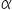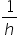b =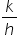……(1)where k is a proportionality constantStep 1 of 2:It is given that when the base is 18 cm long, the height is 7 cm i.e. b = 18 and h = 7. Putting the values in equation (1)18 =k = 18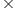7  = 126Step 2 of 2:Now we are asked to determine the length of the base if the height is 6 cm. So, h = 6 and we need to find b. Putting the values in equation (1)b =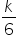Now, put the value of k = 126b =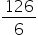b = 21 cmFinal Answer: Hence, the length of the base is 21 cm if the height is 6 cmNote: We can also solve this by equating the area of the triangle in both cases.#### With Turito Foundation.#### Get an Expert Advice From Turito.